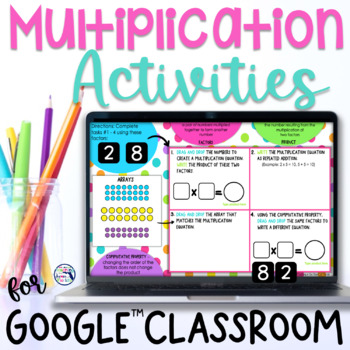# Multiplication Practice | Multiplication Digital Activity

Rated 5 out of 5, based on 2 reviews
2 Ratings;
3rd - 4th, Homeschool
Subjects
Standards
Formats Included
• PDF
•Google Apps™
Pages
22 pages
Report this resource to TPTThe Teacher-Author indicated this resource includes assets from Google Workspace (e.g. docs, slides, etc.).

### Description

This fun digital multiplication activity gives your 3rd grade students 4 different ways to represent multiplication! Repeated practice with multiplication equations, arrays, repeated addition, and the Commutative Property will help them master this third grade math concept!

This digital activity is perfect for an introductory whole class multiplication lesson, math centers, independent practice or homework.

★ Please see preview for a closer look! ★

───────────────────────────

What's Included in this Multiplication Resource:

───────────────────────────

Common Core Math Standards addressed in this resource:
OA.A.1 - Interpret products of whole numbers, e.g., interpret 5 × 7 as the total number of objects in 5 groups of 7 objects each.
OA.B5 - Apply properties of operations as strategies to multiply and divide.

───────────────────────────
Need Multiplication Resources?

Multiplication Worksheets | Multiplication Facts 1-12 | 3rd Grade

───────────────────────────

"Don't forget to leave feedback -you will receive TPT credits that you can use toward future purchases!

Thank you for visiting Teaching in the Heart of Florida!

Total Pages
22 pages
Included
Teaching Duration
Other
Report this resource to TPT
Reported resources will be reviewed by our team. Report this resource to let us know if this resource violates TPT’s content guidelines.

### Standards

to see state-specific standards (only available in the US).
Interpret products of whole numbers, e.g., interpret 5 × 7 as the total number of objects in 5 groups of 7 objects each. For example, describe a context in which a total number of objects can be expressed as 5 × 7.
Apply properties of operations as strategies to multiply and divide. Examples: If 6 × 4 = 24 is known, then 4 × 6 = 24 is also known. (Commutative property of multiplication.) 3 × 5 × 2 can be found by 3 × 5 = 15, then 15 × 2 = 30, or by 5 × 2 = 10, then 3 × 10 = 30. (Associative property of multiplication.) Knowing that 8 × 5 = 40 and 8 × 2 = 16, one can find 8 × 7 as 8 × (5 + 2) = (8 × 5) + (8 × 2) = 40 + 16 = 56. (Distributive property.)